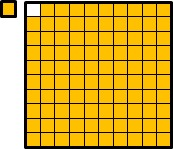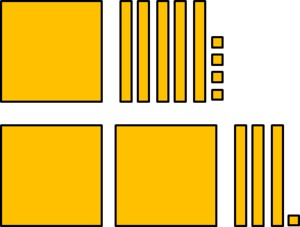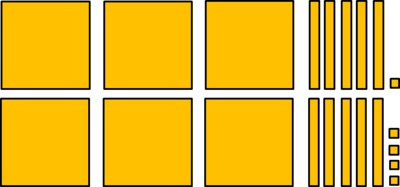# Using Pictures to Explain Number Comparisons

Alignments to Content Standards: 2.NBT.A.4

Compare each pair of numbers. Write your comparison using $\lt, =,$ or $\gt$ and in words. Explain your answer with a picture.

1. 99 and 100
2. 154 and 231
3. 453 and 428
4. 351 and 354

## IM Commentary

The purpose of this task is for students to compare three-digit numbers and explain the comparisons based on the meaning of the hundreds, tens, and ones digits, using >, =, and < symbols to record the results of comparisons. It is important that students not only understand the correct symbol, but that they also understand the words that match the symbols.

The first comparison is between 99 and 100, which is important for two reasons. Understanding this comparison is the key to understanding the rule for comparing three digit numbers depends on understanding that the largest possible two-digit number is less than the smallest possible 3-digit number. The reason this is true is that whenever we have 10 ones, we bundle to make 1 ten, and whenever we have 10 tens, we bundle to make 1 hundred. This allows us to first compare the hundreds place, and then if they are the same we compare the tens place, and then if they are the same we compare the ones place. The other three comparisons are strategically chosen. The second pair has different digits in the hundreds place but the larger number has smaller digits in the tens and ones places. The third pair has the same hundreds digit and different tens digit, while the larger of the two has a smaller digit in the ones place. This assures that students are focused on the meaning of the digits in the hundreds, tens, and ones place.

Students could benefit from working in pairs, especially if they are just getting started understanding the meaning of the hundreds digit. In that case, students might also benefit from having base-ten blocks or bundles of popsicle sticks. In any case, students will probably need some guidance about how to draw the pictures. They can draw pictures like those shown in the solution that look like base-ten blocks, or they can draw squares for the hundreds, lines for the tens, and dots for the ones to make it easier to draw the blocks. Alternatively, they can draw ten quick lines with a circle around it for a bundle of 10 popsicle sticks and lines for singles.

See the attached student printable, which has a bit more scaffolding and could be used for independent practice.

## Attached Resources

• student printable
• ## Solution

1. $99 \lt 100$ which means that 99 is less than 100. If we add one more to 99, the 1 will make a bundle of 10 ones with the 9 ones in 99. Then the 10 tens will make a bundle of 1 hundred. So 99 is one less than 100.2. $154 \lt 231$ which means that 154 is less than 231. 231 has two hundreds, and 154 is less than 200 because it has 1 hundred and some tens and ones that are less than 100 all together.3. $453 \gt 428$ which means that 453 is greater than 428. Both of these numbers have the same number of hundreds. We know $53 > 28$ because 53 has 5 tens and 28 has 2 tens and some ones that total less than ten.4. $351 \lt 354$ which means that 351 is less than 354. Both have the same number of hundreds and tens, but 354 has more ones.